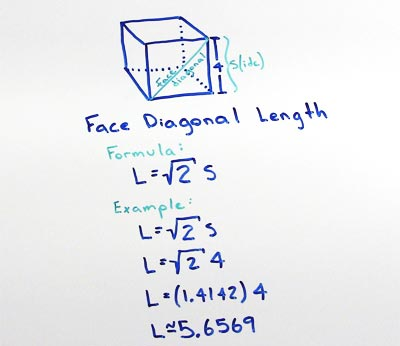# Volume of Cube

## Cube Face Diagonal

A cube with a side (S) of 20 has a maximum face diagonal length (L) of 28.2843.

## Cube Face Diagonal Formula

find the maximum face diagonal Length for cubes with a side length S

• L = S√2

substitute the side length S with the measured value, in this example lets use a side length of 4

• L = 4√2

now use the square root function on your calculator to find the square root of 2, then multiply this by the side length of 4.

• L = 5.6569The longest diagonal line across the face of a cube with side length (S) can be determined by dividing the Side by √2.

### Formulas

What is the formula for the volume of a Cube?

V = S3

What is the formula for the volume of a Rectangular Prism?

V = L x W x H

### Other Formulae

for cubes with side length S

Cube Surface Area

6S2
Cube Face Diagonal
(√2)S
Cube Space Diagonal
(√3)S
S/2
All formulae...